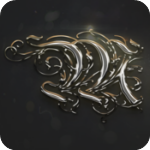# 魔塔Mac版(附攻略)v1.0.2

8.5分### 游戏背景### 魔塔Mac版控制

1、使用箭头键来控制角色。
2、按M静音/播放背景音乐。
3、点击按钮，如果它们是可点击的。
4、加载和保存游戏可用，但只能加载最新的保存。### 游戏攻略

1-2楼：刚上来是按照预设剧情丢掉600血和兵器防具，行走路线只有一条，所以无话可说。
3楼：和小偷一起越狱之后，直接上3楼，再上中吃完瓶子和钥匙[生命=1000，攻击力=10，防御力=10，金钱=4]，向右打绿色史莱姆[生命=976，金钱=5]，找老头要手册，然后向下打红色史莱姆[生命=926，金钱=7]，开门上楼。
4楼：过黄门，直接向左，打绿色史莱姆[生命=902，金钱=8]，打红色史莱姆[生命=852，金钱=10]，开门上楼。
5楼：向上打绿色史莱姆[生命=828，金钱=11]，向右打绿色史莱姆[生命=804，金钱=12]，向下打红色史莱姆[生命=754，金钱=14]，开门拿剑[生命=754，攻击力=20，防御力=10，金钱=14]。向上打红色史莱姆[生命=734，金钱=16]，不过门，回到中路打蝙蝠拿钥匙[生命=678，金钱=19]，下楼。
4楼：直接过黄门打红色史莱姆[生命=658，金钱=21]，再过蓝门去商店，升级防御[生命=658，攻击力=20，防御力=14，金钱=1]。上楼。
5楼：到右上角取钥匙，两个绿色史莱姆只打一个[生命=654]。到中路过门打红色史莱姆[生命=6 42]，打蝙蝠[生命=594]，开门上楼。
6楼：向下右转打红色史莱姆[生命=582]，打初级法师[生命=510]，拿钥匙，向上左转，进门打红色史莱姆[生命=498]拿钥匙，然后向下过两个门，打红色史莱姆[生命=486]补血[生命=586]，过门红色史莱姆[生命=574]，上楼。
7楼：向上出门，左转走到初级法师面前向下，打蝙蝠拿钥匙[生命=526]，打初级法师[生命=454]，打骷髅战士[生命=226]，开门上楼。
8楼：向下开门吃瓶[生命=276]打红/绿/红色史莱姆[生命=248]，向下开门右转打骷髅[生命=16 4]，向上打蝙蝠[生命=116]，再向上打初级法师[生命=44]，拿钥匙开门上楼。
9楼：向右开门打绿色史莱姆[生命=40]，吃瓶[生命=90]，向下走到两扇门前再向下，从漏洞取盾[生命=90，攻击力=20，防御力=24，金钱=56]，回头一直下到5楼。
5楼：过门向下打蝙蝠[生命=62]再回到9楼。
9楼：过两个黄门拿钥匙和红宝石[生命=62，攻击力=21，防御力=24，金钱=59]，再向左过黄门，打蝙蝠[生命=48]，拿钥匙和蓝宝石[生命=48，攻击力=21，防御力=25，金钱=62]，回头一直下到7楼。
7楼：在上楼梯边上那一竖列，开门，打蝙蝠[生命=35]，吃瓶拿红宝石[生命=85，攻击力=22，防御力=25，金钱=65]，然后一直下到3楼。
3楼：去左上角，打蝙蝠[生命=72]，向上过门打初级法师[生命=44]，拿钥匙、蓝宝石和血瓶[生命=94，攻击力=22，防御力=26，金钱=73]，然后上4楼。
4楼：去左上角，打初级法师[生命=70]，吃血拿黄/蓝钥匙[生命=120]，上8楼。
8楼：去左下角打蝙蝠[生命=108]，过黄门打绿色史莱姆[不伤]，打蝙蝠[生命=96]，过蓝门拿钥匙和红/蓝宝石[生命=96，攻击力=23，防御力=27，金钱=85]，下到1楼。
1楼：去右中附近，过黄门打蝙蝠[生命=85]，打初级法师[生命=70]，打蝙蝠[生命=59]，进黄门拿钥匙、血瓶和红/蓝宝石[生命=109，攻击力=24，防御力=28，金钱=96]，然后去4楼。
4楼：去左下过黄门，打蝙蝠[生命=99]拿2把钥匙，去6楼。
6楼：去左下打骷髅[生命=71]，打绿色史莱姆[不伤]，过黄门打蝙蝠[生命=61]，拿蓝宝石并和老头说话[生命=61，攻击力=24，防御力=29，金钱=109]，回头下到3楼。
3楼：去左中过黄门打骷髅[生命=35]，拿钥匙、血瓶和红宝石[生命=85，攻击力=25，防御力=29，金钱=115]，上到4楼。
4楼：下中偏右，过黄门打初级法师[生命=76]，拿红宝石和血瓶[生命=126，攻击力=26，防御力=29，金钱=120]，上到5楼。
5楼：左下方向打骷髅战士[生命=57]，拿钥匙、蓝宝石、血瓶和记事本[生命=107，攻击力=26，防御力=30，金钱=128]，然后下到4楼。
4楼：到左下打绿色史莱姆[不伤]，拿钥匙，下到1楼。
1楼：左中方向过黄门吃瓶打骷髅[生命=133]，向下过门拿钥匙打骷髅战士[生命=67]，过黄门拿血瓶[生命=167]、升降梯和钥匙，然后上到7楼。
7楼：右数第2列完全打通，向上打骷髅[生命=143]，拿血瓶和钥匙[生命=193]，向下打骷髅战士[生命=127]，拿血瓶和钥匙[生命=327]，然后上到9楼。
9楼：沿路向下中走，打红色史莱姆[不伤]，打蝙蝠[生命=319]，过黄门向左，向上打骷髅[生命=295]，过黄门拿钥匙打骷髅战士[生命=229]，过黄门拿钥匙打骷髅战士[生命=163]，过黄门拿血瓶上楼。
10楼：过黄门打骷髅[生命=189]，拿蓝宝石[生命=189，攻击力=26，防御力=31，金钱=190]，向下过黄门打两个初级法师[生命=183]，过黄门打骷髅[生命=161]，拿红宝石[生命=161，攻击力=27，防御力=31，金钱=206]。到此为止，第一关升级完成，下面是补点血准备过关了。目前过关需要530的血[6个骷髅+2个骷髅战士+骷髅队长=6×22+2×63+272=530]。所以先去找血，下到9楼。
9楼：右下角过黄门打初级法师[生命=158]，拿血瓶和钥匙[生命=208]，然后下到4楼。
4楼：去右上打骷髅[生命=186]，过黄门打骷髅战士[生命=123]，拿血瓶和钥匙[生命=323]，和老头说话，然后上到8楼。
8楼：右中偏下打初级法师[生命=320]，过黄门打两个初级卫兵[生命=14]，拿血瓶和钥匙[生命=26 4]，然后下到7楼。
7楼：右数第3列下方，过黄门打红色史莱姆[不伤]，打初级法师[生命=261]，拿血瓶[生命=461]，然后下到1楼。
1楼：右下角过黄门打蝙蝠[生命=454]，拿血瓶[生命=654]，然后上10楼。
10楼：决斗开始，一场屠杀之后胜利[生命=124]，拿战利品红/蓝宝石、钥匙和1个血瓶[生命=324，攻击力=30，防御力=34，金钱=346]。

### 更新日志

v1.0.2

mac好玩的游戏 共收集10款软件

0条评论